# Medical Image-Based Finite Element Analysis of Spine

This example shows how to estimate bone stress and strain in a vertebra bone under axial compression using finite element (FE) analysis.

Bones experience mechanical loading due to gravity, motion, and muscle contraction. Estimating the stresses and strains within bone tissue can help predict bone strength and fracture risk. This example uses image-based FE analysis to predict bone stress and strain within a vertebra under axial compression. Image-based FE uses medical images, such as computed tomography (CT) scans, to generate the geometry and material properties of the FE model.

In this example, you create and analyze a 3-D model of a single vertebra under axial loading using this workflow:

This example uses a CT scan saved as a directory of DICOM files. The scan is part of a data set that contains three CT volumes. Run this code to download the data set from the MathWorks website as a zip file and unzip the file. The total size of the data set is approximately 81 MB.

```zipFile = matlab.internal.examples.downloadSupportFile("medical","MedicalVolumeDICOMData.zip"); filepath = fileparts(zipFile); unzip(zipFile,filepath)```

After you download and unzip the data set, the scan used in this example is available in the `dataFolder` directory.

`dataFolder = fullfile(filepath,"MedicalVolumeDICOMData","LungCT01");`

### Segment Vertebra from CT Scan

This example uses a binary segmentation mask of one vertebra in the spine. The mask was created by segmenting the spine from a chest CT scan using the Medical Image Labeler app. For an example of how to segment medical image volumes in the app, see Label 3-D Medical Image Using Medical Image Labeler.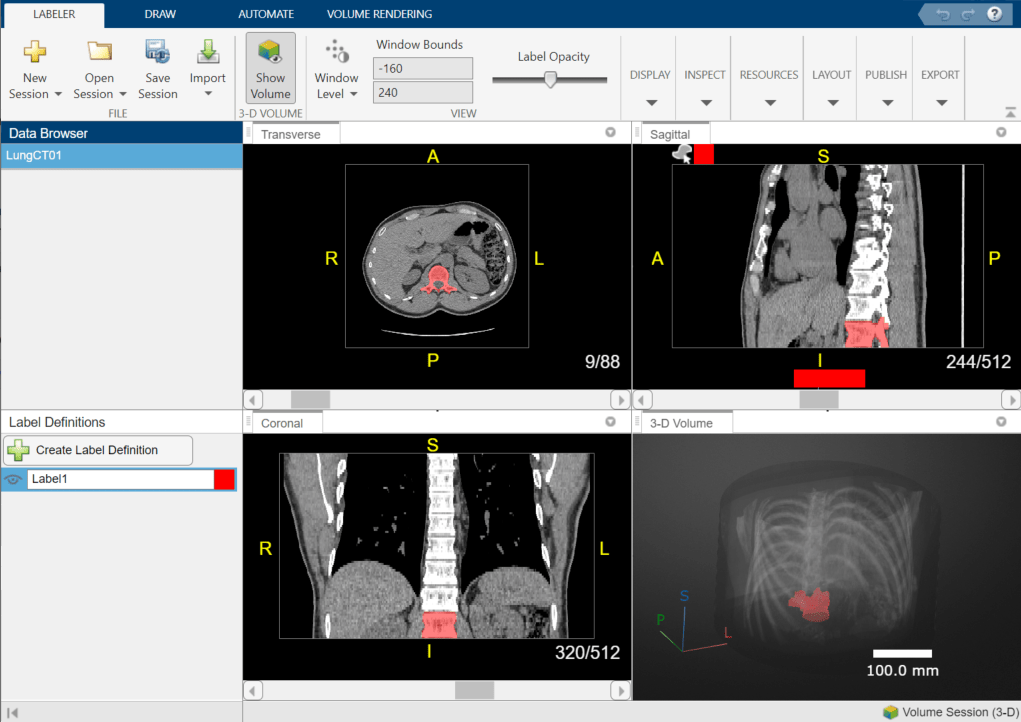The segmentation mask is attached to this example as a supporting file. Load the mask as a `medicalVolume` object.

`labelVol = medicalVolume("LungCT01.nii.gz");`

The `VolumeGeometry` property of a medical volume object contains a `medicalref3d` object that defines the patient coordinate system for the scan. Extract the `medicalref3d` object for the mask into a new variable, `R`.

`R = labelVol.VolumeGeometry;`

### Extract Vertebra Geometry and Generate FE Mesh

#### Extract Isosurface of Vertebra Mask

Calculate the isosurface faces and vertices for the vertebra mask by using the `extractIsosurface` function. The `vertices` coordinates are in the intrinsic coordinate system defined by rows, columns, and slices.

```isovalue = 1; [faces,vertices] = extractIsosurface(labelVol.Voxels,isovalue);```

Transform `vertices` to the patient coordinate system defined by `R` by using the `intrinsicToWorld` object function. The `X`, `Y`, and `Z` outputs define the xyz-coordinates of the surface points, in millimeters.

```I = vertices(:,1); J = vertices(:,2); K = vertices(:,3); [X,Y,Z] = intrinsicToWorld(R,I,J,K); verticesPatient = [X Y Z];```

Convert the vertex coordinates to meters.

`verticesPatientMeters = verticesPatient.*10^-3;`

Display the vertebral isosurface as a triangulated `Surface` object.

```triangul = triangulation(double(faces),double(verticesPatientMeters)); viewer = viewer3d; surface = images.ui.graphics3d.Surface(viewer,data=triangul,Color=[1 0 0],Alpha=1);```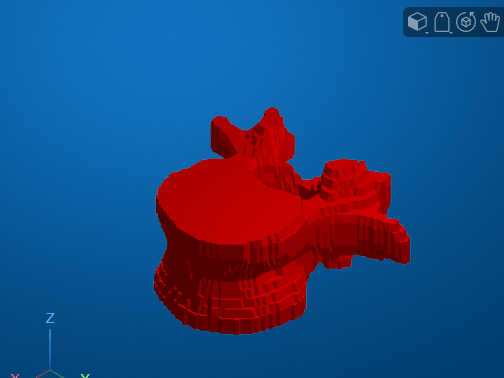#### Create PDE Model Geometry

Create a general `PDEModel` model container for a system of three equations. A general model allows you to assign spatially varying material properties based on bone density.

`model = createpde(3);`

Specify the geometry for the model by using the `geometryFromMesh` (Partial Differential Equation Toolbox) object function, with the isosurface in patient coordinates as input.

```geometryFromMesh(model,verticesPatientMeters',faces'); pdegplot(model,FaceLabels="on")```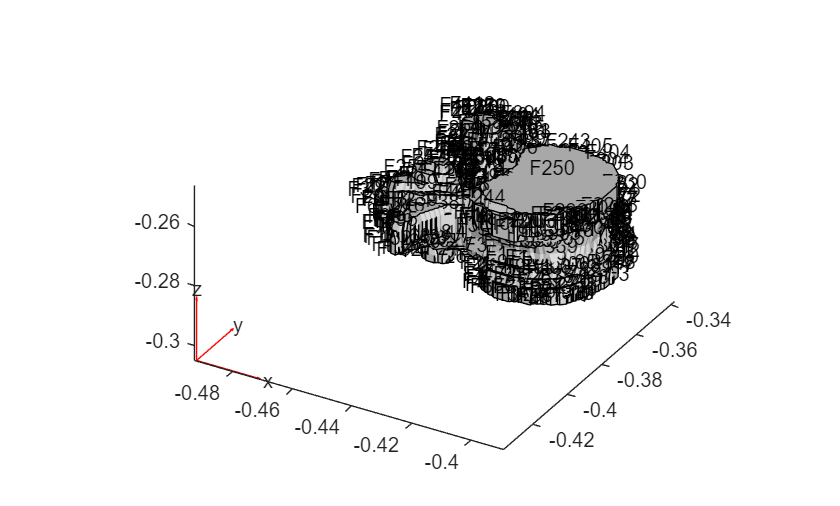#### Generate Mesh from Model Geometry

Specify the maximum edge length for the FE mesh elements. This example uses a max edge length of 1.6 mm, which was determined using a mesh convergence analysis.

`hMax = 0.0016;`

Generate the mesh from the model geometry. Specify the maximum edge length, and specify that the minimum edge length is half of the maximum edge length. The elements are quadratic tetrahedra.

```msh = generateMesh(model,Hmax=hMax,Hmin=hMax/2); pdemesh(model);```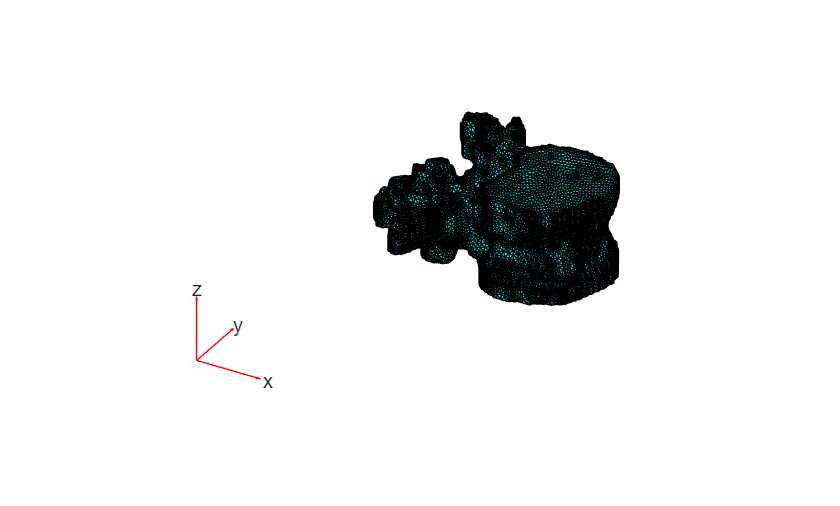### Assign Material Properties

Bone material properties vary based on spatial location and direction:

• Spatial location — Local regions with greater bone density are stiffer than low density regions.

• Direction — Bone stiffness depends on the loading direction. This example models bone using transverse isotropy. A transversely isotropic material is stiffer in the axial direction versus in the transverse plane, and is uniform within the transverse plane.

In this example, you assign spatially varying, transversely isotropic linear elastic material properties.

#### Extract HU Values Within Vertebra Mask

To assign spatially varying material properties, you need to map CT intensity values to FE mesh coordinates.

Load the original, unsegmented CT scan using a `medicalVolume` object. The `medicalVolume` object automatically converts the intensity to Hounsfield units (HU). The HU value is a standard for measuring radiodensity.

`DCMData = medicalVolume(dataFolder);`

Extract the indices and intensities of CT voxels inside the vertebra mask.

```trueIdx = find(labelVol.Voxels==1); HUVertebra = double(DCMData.Voxels(trueIdx));```

Convert the linear indices in `trueIdx` into subscript indices, in units of voxels.

`[row,col,slice] = ind2sub(size(labelVol.Voxels),trueIdx);`

Transform the subscript indices into patient coordinates and convert the coordinates from millimeters to meters.

```[X2,Y2,Z2] = intrinsicToWorld(R,col,row,slice); HUVertebraMeters = [X2 Y2 Z2].*10^-3;```

#### Map HU Values from CT Voxels to Mesh Nodes

The FE node coordinates are scattered and not aligned with the CT voxel grid. Create a `scatteredInterpolant` object to define the 3-D interpolation between the voxel grid and FE nodes.

`F = scatteredInterpolant(HUVertebraMeters,HUVertebra);`

#### Specify Coefficients

Specify the PDE coefficients for the model. In this structural analysis, the c coefficient defines the material stiffness of the model, which is inversely related to compliance. To define a spatially varying c coefficient in Partial Differential Equation Toolbox™, represent `c` in function form. In this example, the `HU2TransverseIsotropy` helper function defines transversely isotropic material properties based on bone density. Bone density is calculated for a given location using the scattered interpolant `F`. The helper function is wrapped in an anonymous function, `ccoeffunc`, which passes the `location` and `state` structures to `HU2TransverseIsotropy`. The FE solver automatically computes `location` and `state` structure arrays and passes them to your function during the simulation.

```ccoeffunc = @(location,state) HU2TransverseIsotropy(location,state,F); specifyCoefficients(model,'m',0, ... 'd',0, ... 'c',ccoeffunc, ... 'a',0, ... 'f',[0;0;0]);```

Identify the faces in the model geometry to apply the boundary conditions. In this example, the faces were identified using visual inspection of the plot created above using `pdegplot`.

```bottomSurfaceFace = 1; topSurfaceFace = 250;```

Specify the total force to apply, in newtons.

`forceInput = -3000;`

Estimate the area of the top surface.

```nf2=findNodes(model.Mesh,"region",Face=topSurfaceFace); positions = model.Mesh.Nodes(:,nf2)'; surfaceShape = alphaShape(positions(:,1:2)); faceArea = area(surfaceShape);```

Calculate the pressure magnitude as a function of force and area, in pascals.

`inputPressure_Pa = forceInput/faceArea;`

Apply the boundary conditions.

```applyBoundaryCondition(model,"dirichlet",Face=bottomSurfaceFace,u=[0,0,0]); applyBoundaryCondition(model,"neumann",Face=topSurfaceFace,g=[0;0;inputPressure_Pa]);```

Solve the model. The output is a `StationaryResults` (Partial Differential Equation Toolbox) object that contains nodal displacements and their gradients.

`Rs = solvepde(model);`

### Analyze Results

View the results of the simulation by plotting the axial displacement, stress, and strain within the model.

#### Displacement

Plot axial displacement, in millimeters, for the full model by using the `pdeplot3D` function.

```Uz = Rs.NodalSolution(:,3)*10^3; pdeplot3D(model,"ColorMapData",Uz) clim([-0.15 0]) title("Axial Displacement (mm)")```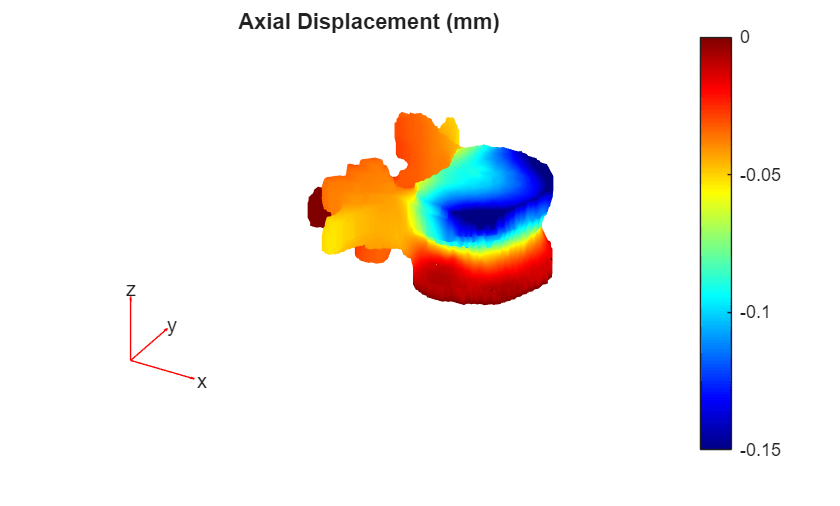Plot displacement in a transverse slice at the midpoint of vertebral height.

Create a rectangular grid that covers the geometry of the transverse (xy) slice with spacing of 1 mm in each direction. The `x` and `y` vectors define the spatial limits and spacing in the transverse plane, and `z` is a scalar the provides the midpoint of vertebral height. The `Xg`, `Yg`, and `Zg` variables define the grid coordinates.

```x = min(msh.Nodes(1,:)):0.001:max(msh.Nodes(1,:)); y = min(msh.Nodes(2,:)):0.001:max(msh.Nodes(2,:)); z = min(msh.Nodes(3,:))+0.5*(max(msh.Nodes(3,:))-min(msh.Nodes(3,:))); [Xg,Yg] = meshgrid(x,y); Zg = z*ones(size(Xg));```

Interpolate normal axial displacement onto the grid coordinates by using the `interpolateSolution` (Partial Differential Equation Toolbox) object function. Convert displacement from meters to millimeters, and reshape the displacement vector to the grid size.

```U = interpolateSolution(Rs,Xg,Yg,Zg,3); U = U*10^3; Ug = reshape(U,size(Xg));```

Plot axial displacement in the transverse slice.

```surf(Xg,Yg,Ug,LineStyle="none") axis equal grid off view(0,90) colormap("turbo") colorbar clim([-0.15 0]) title("Axial Displacement (mm)")```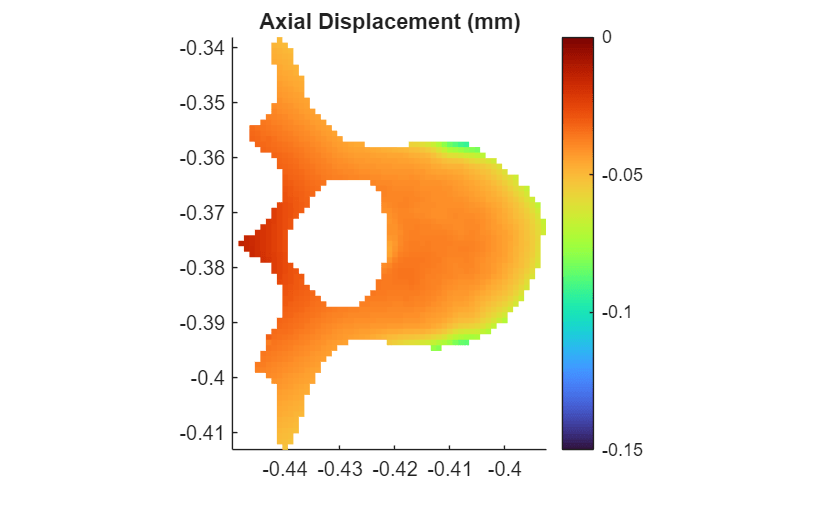#### Stress

In a structural analysis, stress is the product of the c coefficient and the gradients of displacement. Calculate normal stresses at the transverse slice grid coordinates by using the `evaluateCGradient` (Partial Differential Equation Toolbox) object function.

`[cgradx,cgrady,cgradz] = evaluateCGradient(Rs,Xg,Yg,Zg,3);`

Convert normal axial stress to megapascals, and reshape the stress vector to the grid size.

```cgradz = cgradz*10^-6; cgradzg = reshape(cgradz,size(Xg));```

Plot the normal axial stress in the transverse slice.

```surf(Xg,Yg,cgradzg,LineStyle="none"); axis equal grid off view(0,90) colormap("turbo") colorbar clim([-10 1]) title("Axial Stress (MPa)")```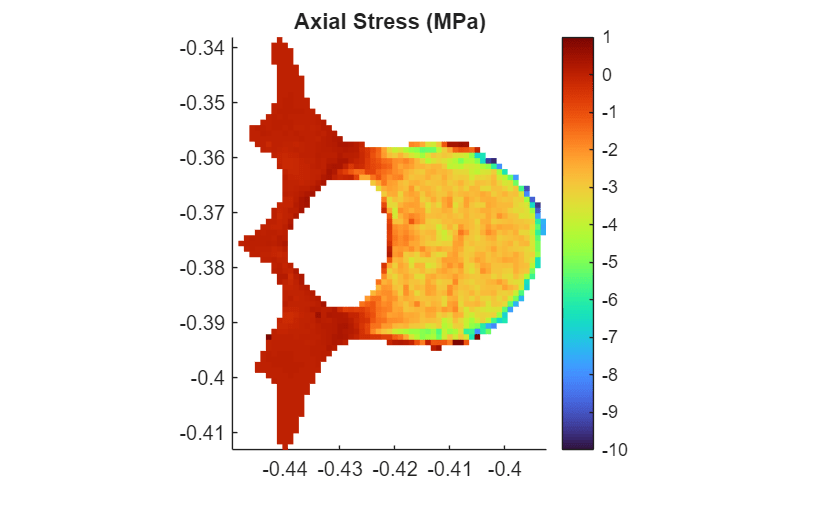#### Strain

In a structural analysis, strain is the gradient of the displacement result. Extract the normal strain at the transverse slice grid coordinates by using the `evaluateGradient` (Partial Differential Equation Toolbox) object function.

`[gradx,grady,gradz] = evaluateGradient(Rs,Xg,Yg,Zg,3);`

Reshape the axial strain vector to the grid size.

`gradzg = reshape(gradz,size(Xg));`

Plot the axial strain in the transverse slice.

```surf(Xg,Yg,gradzg,LineStyle="none"); axis equal grid off view(0,90) colormap("turbo") colorbar clim([-0.008 0]) title("Axial Strain")```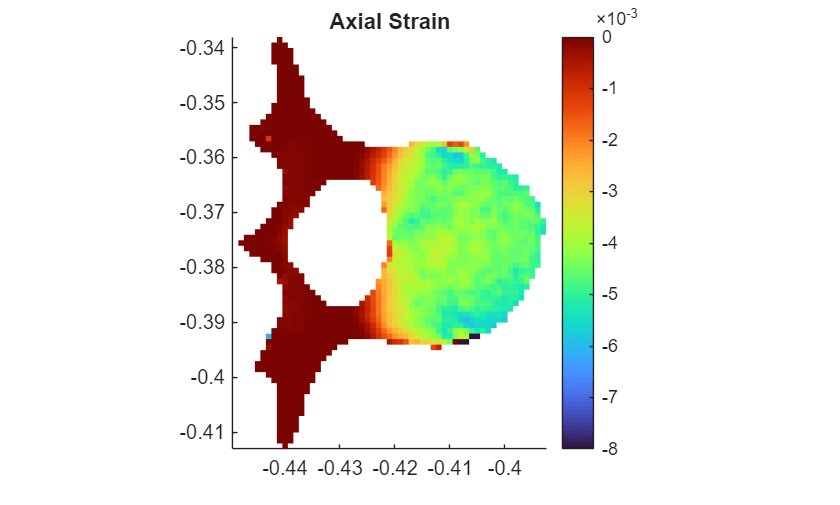### Helper Functions

The `HU2TransverseIsotropy` helper function specifies transverse isotropic material properties using these steps:

• Map voxel intensity to Hounsfield units (HU) at nodal locations using the `scatteredInterpolant` object, `F`.

• Convert HU to CT density using a linear calibration equation from .

• Convert CT density to elastic modulus in the axial direction, `E3`, and in the transverse plane, `E12`, as well as the bulk modulus, `G`, using density-elasticity relationships from . The Poisson's ratio in the axial direction, `v3`, and transverse plane, `v12`, are also assigned based on .

• Define the 6-by-6 compliance matrix for transverse isotropy by using the `elasticityTransOrthoC3D` helper function.

• Convert the 6-by-6 compliance, or c-matrix, to the vector form required by Partial Differential Equation Toolbox by using the `SixMat2NineMat` and `SquareMat2CCoeffVec` helper functions.

```function ccoeff = HU2TransverseIsotropy(location,state,F) HU = F(location.x,location.y,location.z); rho = 5.2+0.8*HU; E3 = -34.7 + 3.230.*rho; E12 = 0.333*E3; v12 = 0.104; v3 = 0.381; G = 0.121*E3; ccoeff = zeros(45,length(location.x)); for i = 1:length(location.x) cMatrix = elasticityTransOrthoC3D(E12(i),E3(i),v12,v3,G(i)); nineMat = SixMat2NineMat(cMatrix); ccoeff(:,i) = SquareMat2CCoeffVec(nineMat); end end```

The `elasticityTransOrthoC3D` helper function defines the 6-by-6 compliance matrix for a transversely isotropic linear elastic material based on the elastic moduli, `E12` and `E3`, bulk modulus, `G`, and Poisson's ratios, `v12` and `v3`. The helper function converts all modulus values from megapascals to pascals before computing the compliance matrix.

```function C = elasticityTransOrthoC3D(E12,E3,v12,v3,G) E12 = E12*10^6; E3 = E3*10^6; G = G*10^6; v_zp = (E3*v3)/E12; C = zeros(6,6); C(1,1) = 1/E12; C(1,2) = -v12/E12; C(1,3)= -v_zp/E3; C(2,1) = C(1,2); C(2,2) = 1/E12; C(2,3) = -v_zp/E3; C(3,1) = -v3/E12; C(3,2) = C(2,3); C(3,3) = 1/E3; C(4,4) = 1/(2*G); C(5,5) = 1/(2*G); C(6,6) = (1+v12)/E12; C=inv(C); end```

The `SixMat2NineMat` helper function converts a 6-by-6 c coefficient matrix in Voigt notation to a 9-by-9 matrix corresponding to expanded form.

```function nineMat = SixMat2NineMat(sixMat) for i = 1:6 nineVecs(i,:) = sixMat(i,[1 6 5 6 2 4 5 4 3]); end nineMat = [ nineVecs(1,:); ... nineVecs(6,:); ... nineVecs(5,:); ... nineVecs(6,:); ... nineVecs(2,:); ... nineVecs(4,:); ... nineVecs(5,:); ... nineVecs(4,:); ... nineVecs(3,:)]; end```

The `SquareMat2CCoeffVec` converts a 9-by-9 c coefficient matrix into vector form as required by Partial Differential Equation Toolbox. This helper function creates a 45-element vector, corresponding to the "3N(3N+1)/2-Element Column Vector c, 3-D Systems" case in c Coefficient for specifyCoefficients (Partial Differential Equation Toolbox).

```function c45Vec = SquareMat2CCoeffVec(nineMat) C11 = [nineMat(1,1) nineMat(1,2) nineMat(2,2) nineMat(1,3) nineMat(2,3) nineMat(3,3)]; C12 = [nineMat(1,4) nineMat(2,4) nineMat(3,4) nineMat(1,5) nineMat(2,5) nineMat(3,5) ... nineMat(1,6) nineMat(2,6) nineMat(3,6)]; C13 = [nineMat(1,7) nineMat(2,7) nineMat(3,7) nineMat(1,8) nineMat(2,8) nineMat(3,8) ... nineMat(1,9) nineMat(2,9) nineMat(3,9)]; C22 = [nineMat(4,4) nineMat(4,5) nineMat(5,5) nineMat(4,6) nineMat(5,6) nineMat(6,6)]; C23 = [nineMat(4,7) nineMat(5,7) nineMat(6,7) nineMat(4,8) nineMat(5,8) nineMat(6,8) ... nineMat(4,9) nineMat(5,9) nineMat(6,9)]; C33 = [nineMat(7,7) nineMat(7,8) nineMat(8,8) nineMat(7,9) nineMat(8,9) nineMat(9,9)]; c45Vec = [C11 C12 C22 C13 C23 C33]'; end```

### References

 Turbucz, Mate, Agoston Jakab Pokorni, György Szőke, Zoltan Hoffer, Rita Maria Kiss, Aron Lazary, and Peter Endre Eltes. “Development and Validation of Two Intact Lumbar Spine Finite Element Models for In Silico Investigations: Comparison of the Bone Modelling Approaches.” Applied Sciences 12, no. 20 (January 2022): 10256. https://doi.org/10.3390/app122010256.

 Unnikrishnan, Ginu U., and Elise F. Morgan. “A New Material Mapping Procedure for Quantitative Computed Tomography-Based, Continuum Finite Element Analyses of the Vertebra.” Journal of Biomechanical Engineering 133, no. 7 (July 1, 2011): 071001. https://doi.org/10.1115/1.4004190.